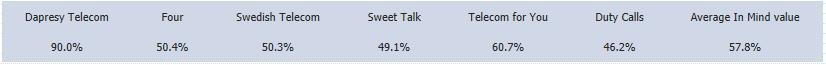There is an option for adding mean value series to a table based on the shown result. The feature can be used in a lot of different areas and project types. Below are two examples. Example 1: A brand tracker project. The table shows an “In Mind” question and a Mean value series has been added to show the mean value of all the brands.
The ”Average in Mind Value” column in the table below has been created by using the new Mean value series option.Example 2: A customer satisfaction project. The table shows the result of a question, one column shows the result of the selected country and the other column shows a Mean value series based on the “Top 5 Countries” in the company. The “Mean Value of Top 5 Countries” in the table below has been created by using the new Mean value series option. (Two separate table objects have been used: one for the column, Denmark, and one for the column, Mean value top 5 countries).The mean value series can be shown together with the original series or on its own. The Mean value series can consider the base sizes in the original series or only be based on the calculated values.

The setup of the Mean Value series is made in the Table setup window in the Analysis stab. The following options are defined during the setup:

• Should the Mean value series be added to the rows or columns
• Should the calculation of the Mean value series consider the base size of the in going series or not
• Should the original series be shown or not
• Should the Mean value series be added as the first or last series in the table
• What should the label of the Mean value series be

The image below shows the setup interface.The value of the Mean value series is the mean value of the series shown in the table. The base size of the original series is only considered if the option “Consider Base Size in Calculation” has been enabled. Series that are hidden due to low base sizes, etc…, are not included in the calculation. It is only the series shown in the table that are included in the calculation of the Mean Value series. The Mean Value calculation uses values which are not rounded. Rounding is only made in the last step.